# HSSlive: Plus One & Plus Two Notes & Solutions for Kerala State Board

## AP Board Class 7 Maths Chapter 2 Fractions Decimals and Rational Numbers InText Questions Textbook Solutions PDF: Download Andhra Pradesh Board STD 7th Maths Chapter 2 Fractions Decimals and Rational Numbers InText Questions Book AnswersAP Board Class 7 Maths Chapter 2 Fractions Decimals and Rational Numbers InText Questions Textbook Solutions PDF: Download Andhra Pradesh Board STD 7th Maths Chapter 2 Fractions Decimals and Rational Numbers InText Questions Book Answers

## Andhra Pradesh State Board Class 7th Maths Chapter 2 Fractions Decimals and Rational Numbers InText Questions Books Solutions

 Board AP Board Materials Textbook Solutions/Guide Format DOC/PDF Class 7th Subject Maths Chapters Maths Chapter 2 Fractions Decimals and Rational Numbers InText Questions Provider Hsslive

## How to download Andhra Pradesh Board Class 7th Maths Chapter 2 Fractions Decimals and Rational Numbers InText Questions Textbook Solutions Answers PDF Online?

2. Click on the Andhra Pradesh Board Class 7th Maths Chapter 2 Fractions Decimals and Rational Numbers InText Questions Answers.
3. Look for your Andhra Pradesh Board STD 7th Maths Chapter 2 Fractions Decimals and Rational Numbers InText Questions Textbooks PDF.
4. Now download or read the Andhra Pradesh Board Class 7th Maths Chapter 2 Fractions Decimals and Rational Numbers InText Questions Textbook Solutions for PDF Free.

## AP Board Class 7th Maths Chapter 2 Fractions Decimals and Rational Numbers InText Questions Textbooks Solutions with Answer PDF Download

Find below the list of all AP Board Class 7th Maths Chapter 2 Fractions Decimals and Rational Numbers InText Questions Textbook Solutions for PDF’s for you to download and prepare for the upcoming exams:

Do This

Question 1.
Write five examples, each of proper, improper, mixed fractions. ? (Page No. 27)
Solution:
Proper fractions
15,23,47,38,49

Improper fractions
72,32,94,115,83

Mixed fractions
123,235,417,867

Question 2.
Write five equivalent fractions for. i) 35 ii) 47 (Page No. 28)
Solution: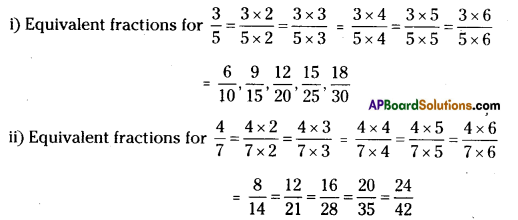Do This

Question 1. (Page No. 31)
i) 4 x 27
ii) 4 x 35
iii) 7 x 13
Solution:
i) 4 x 27=4×27=87
ii) 4 x 35=4×35=125
iii) 7 x 13=7×13=73

Question 2.
Find i) 5 x 32 =
ii) 4 x 75 =
iii) 7 x 83 = (Page No. 31)
Solution:
i) 5 x 32=5×32=152=712
ii) 4 x 75=4×75=285=535
iii) 7 x 83=7×83=563=1823

Question 3.
Find the following: (Page No. 32)
i) 3 x 2 27
ii) 5 x 213
iii) 8 x 417
iv) 4 x 129
v) 5 x 113
Solution: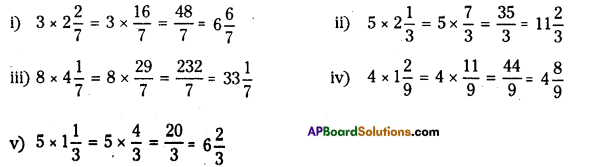Do These

Question 1.
Fill in these boxes. (Page No. 35)
i) 15×17=1×15×7 = ……………
ii) 12×16=1×12×6= = …………………
Solution:
i) 15×17=1×15×7=135
ii) 12×16=1×12×6=112

Do This

Question 1.
Find (Page No. 39)
i) 2 ÷ 14
ii) 7 ÷ 12
iii) 3 ÷ 15 (Page No. 39)
Solution:
i) 2 ÷ 14=2×41=81 = 8
ii) 7 ÷ 12=7×21 = 14
iii) 3 ÷ 15=3×51 = 15

Question 2.
Find (Page No. 41)
i) 9 ÷ 25
ii) 3 ÷ 47
iii) 2 ÷ 89
Solution:
i) 9 ÷ 25 = 9×52=452
ii) 3 ÷ 47 = 3×74=214
iii) 2 ÷ 89 = 2×98=94

Question 3.
Find (Page No. 41)
i) 7 ÷ 513
ii) 5 ÷ 247
Solution:
i) 7 ÷ 513 = 7÷163=7×316=2116
ii) 5 ÷ 247 = 5÷187=5×718=3518

Question 4.
Find (Page No. 42)
i) 35÷12
ii) 12÷35
iii) 212÷35
iv) 516÷92
Solution: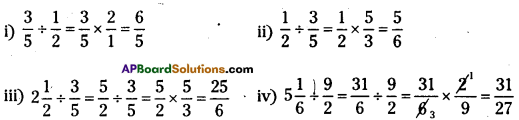Do This

Question
Find (Page No. 45)
i) 0.25 + 5.30
ii) 29.75 – 25.97
Solution:
i) 0.25 + 5.30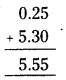ii) 29.75 – 25.97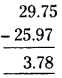Do These

Question 1.
Find (Page No. 48)
i) 1.7 x 3
ii)2.0 x 1.5
iii) 2.3 x 4.35
Solution:
i)1.7 x 3 = 5.1
ii) 2.0 x 1.5 = 3.00
iii) 2.3 x 4.35 = 10.005

Question 2.
Arrange the products obtained in (I) In descending order.
Solution:
Arranging above answers in descending order 10.005 > 5.1 > 3.00

Question 3.
Find (Page No. 50)
i) 35.7 ÷ 3
ii) 25.5 ÷ 3
Solution: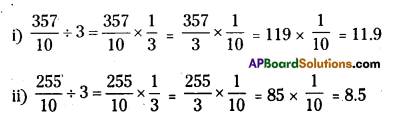Do These

Question 1.
Find the greatest and the smallest numbers among the following groups. (Page No. 52)
i) 2, -2, -3, 4, 0, -5
ii) -3, -7, -8,0,-5,-2
Solution:
i) 2, -2, -3, 4, 0, -5 : greatest number = 4; smallest number = -5
ii) -3, -7, -8, 0, -5, -2: greatest number = 0; smallest number = -8

Question 2.
Write the following numbers In ascending order. (Page No. 52)
i) -5,-75,3,-2,4, 32
ii) 23,32, 0, -1, -2, 5
Solution:
i) -5, -75, 3, -2, 4, 32
Ascending order = -75 <-5 <-2 < 32 <3 < 4 or -75, -5, -2, 32, 3, 4

ii) 23,32, 0, -1, -2, 5
Ascending order = -2, -1, 0, 23, 32, 5

Question 3.
Write 5 equlvalent rational numbers to (i) 52 (Page No. 56)
(ii) −78
(iii) −37
Solution: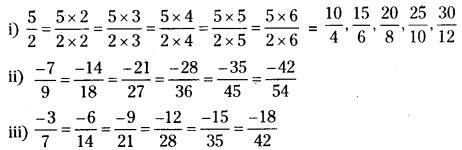Do These

Question 1.
Which is bigger 58 or 35 ? (PageNo.28)
Solution:
58×55=2540 and 35×88=2440
As 2440<2540 58 is bigger than 35

Question 2.
Determine if the following pairs are equal by writing each in their simplest form. (Page No. 28)
i) 38 and 3751000
Solution: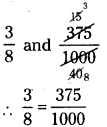ii) 1854 and 2369
Solution: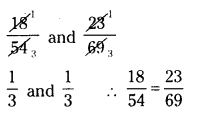iii) 610 and 6001000
Solution: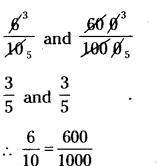iv) 1727 and 2545
Solution”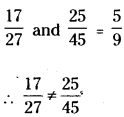Do These

Question 1.
Identify the equivalent rational number is each question
i) −12,−34,−24,−48
Solution:
−12=−24=−48

ii) 14,34,53,106,24,2012
Solution:
53=2012=106

Try This

Question 1.
You have seen that the product of two natural numbers is one or more than one is bigger than each of the two natural numbers. For example 3 x 4 = 12; 12 > 4 and 12 > 3. What happens to the value of the product when we multiply two proper fractions? (Page No.37)
Fill the following table and conclude your observations.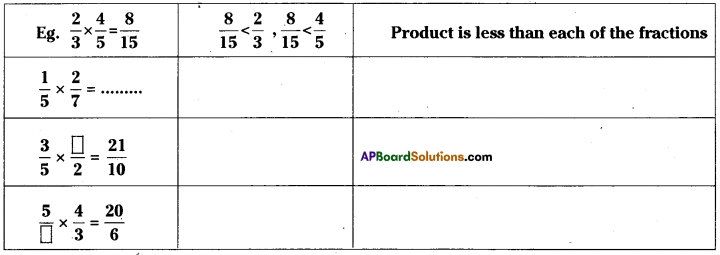Solution: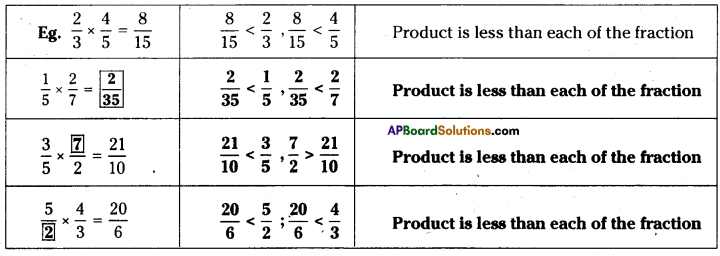Question 2.
Will the reciprocal of a proper fraction be a proper fraction? (Page No. 40)
Solution:
No. Reciprocal of a proper fraction is always an improper fraction.

Question 3.
Will the reciprocal of an Improper fraction be an Improper fraction?
Solution:
No. The reciprocal of an improper fraction is always a proper fraction.

Question 4.
Look at the following table and fill up the blank spaces. (Page No. 44)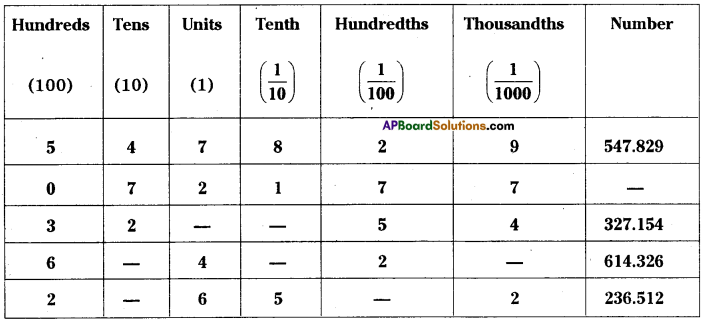Solution: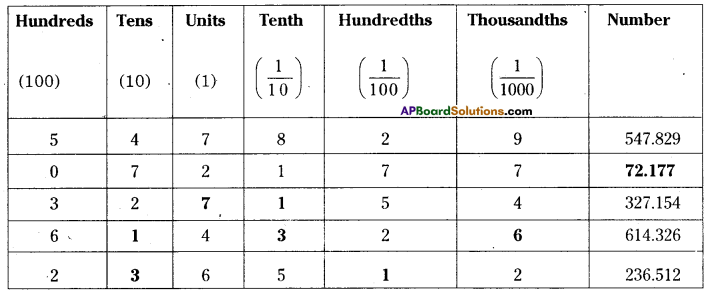Question 5.
WrIte the following numbers in their expanded form. (Page No.44)
i) 30.807
ii) 968.038
iii) 8370. 705
Solution:
i) 30.8O7 = 10 x 3 + 1 x 0 + 110 x 8 +1100 x 0 + 11000 x 7 = 30 + 810+71000

ii) 968.038 = 100 x 9 + 10 x 6 + 1 x 8 + 110 x 0 + 1100 x 3 + 11000 x 8 = 900 + 60 + 8 + 3100+81000

iii) 8370.705 = 1000 x 8 + 100 x 3 + 10 x 7 + 110 x 7 + 11000 x 5 = 8000 + 300 + 70 + 710+51000

Question 6.
Take any5 Integers and make all possible rational numbrs with them. (Page No. 54)
Solution:
Consider 2, 3, 4, 5 and 7
Ratlonalnumberare 23,24,25,27,34,35,37,32,42,43,45,47,52,53,54,57,72,73,74,75

Question 7.
Consider any 5 rational numbers. Find out which itegers constitute them? (Page No.54)
Solution:
Take 34,58,611,27 and 15.
The integers are 1, 2, 3, 4, 5, 6, 7, 8 and 11.

Do This

Question 1.
Find (i) 50 paise = ₹………….. (ii) 22 g = ………….. kg (iii) 80 cm = ………………m (Page No. 44)
Solution:
(i) 50 paise =₹50100 = ₹ 0.5
(ii) 22 g = 221000 kg = 0.022 kg
(iii) 80 cm = 80100 m = 0.8 m

Try This

Question 1.
Represent 34 and 14 in different ways using different figures. Justify your representation. Share, and check it with your friends. (Page No.27)
Solution: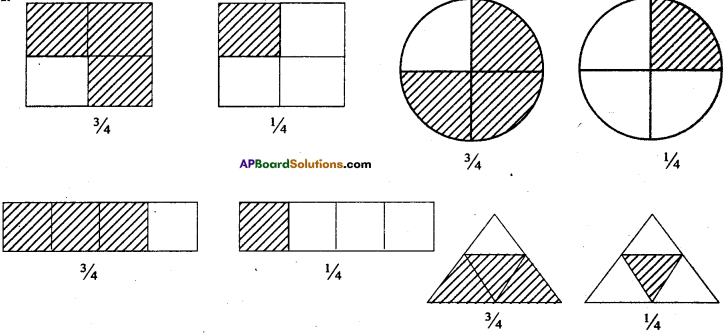Question 2.
Represents 2 1/4 pictorially. How many units are needed for this. (Page No. 27)
Solution: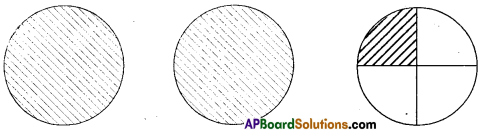We need 3 units to represent 2½

Do These

Question 1.
Represent pictorially 2 x 15=25 (Page No. 32)
Solution: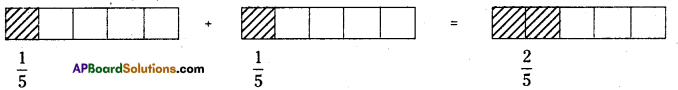Do These

Question 1.
Find 12×15 and 15×12 using diagram check whether 12×15=15×12 (Page No. 35)
Solution: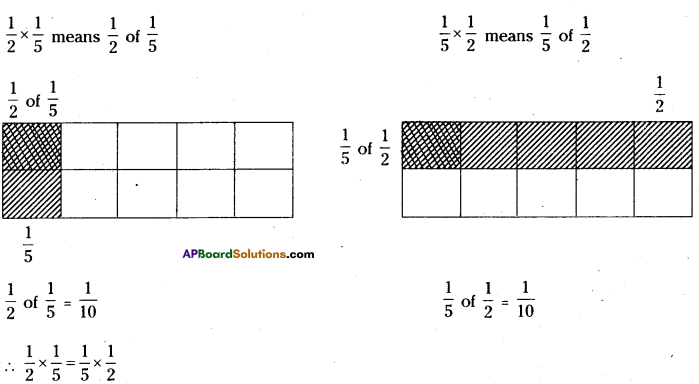Do These

Question 1.
Write 5 more fractions between (i) 0 and 1 (ii) 1 and 2. (Page No. 52)
Solution:
i) Fractions between 0 and 1 are 17,27,37,47,57,67
ii) Fractions between land 2 are 87,97,107,117,127,137

Question 2.
Where does 435 lie on the number line? (Page No. 52)
Solution:
435 lies between 4 and 5 on the number line.

Question 3.
On the number line given below represent the following numbei. (Page No.53)
i) −72
ii) 32
iii) 74
iv) −74
v) −12
vi) 14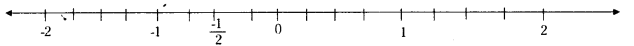Solution: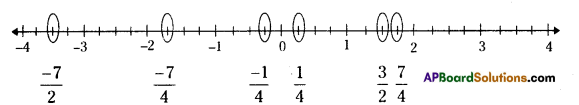Question 4.
Consider the following numbers on a number line. (Page No. 53)
27, −78,11943,5417, -68, -3, −96,72
i) Which of these are to the left of a) 0
Solution:
Left to zero are negative numbers
∴ −78, -68, -3, −96

b) -2
Left to – 2 are less than – 2.
∴ -3, -68

c) 4
Left to 4 are less than 4.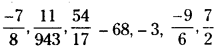d) 2
Left to 2 are less than 2.
∴ −78,11943−68,−3,−96

ii) Which of these would be to the right of
a) 0
Right to zero are positive number.
∴ 27,11943,5417,72

b) -5
Right to -5 are greater than -5.
27,−78,11943,5417−3,−96,72

c) 312
Right to 312 are more than 312.
∴ 27

d) −52
Right to −52 are more than −52
∴ −27,−78,11943,5417−96,72

Try These

Question 1.
Write three more equivalent fractions of 34 and mark them on the number line. What do you observe? (Page No. 55)
Solution:
Equivalent fractions of 34 lie on the same mark.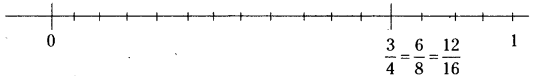Question 2.
Do all equivalent fractions of 67 represent the same point on the number line.
(Page No. 55)
Solution:
Yes.

Question 3.
Are −12 and −36 represent same point on the number line? (Page No. 55)
Solution:
Yes.

Question 4.
Are −23 and −46 equivalent? . (Page No. 55)
Solution:
Yes.

Question 5.
Mark the following rational numbers on the number line. (In Ex 7,3)
(i) 12
(ii) 34
(iii) 32
(iv) 103
Solution: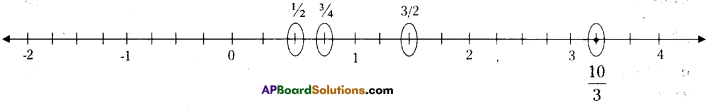## Andhra Pradesh Board Class 7th Maths Chapter 2 Fractions Decimals and Rational Numbers InText Questions Textbooks for Exam Preparations

Andhra Pradesh Board Class 7th Maths Chapter 2 Fractions Decimals and Rational Numbers InText Questions Textbook Solutions can be of great help in your Andhra Pradesh Board Class 7th Maths Chapter 2 Fractions Decimals and Rational Numbers InText Questions exam preparation. The AP Board STD 7th Maths Chapter 2 Fractions Decimals and Rational Numbers InText Questions Textbooks study material, used with the English medium textbooks, can help you complete the entire Class 7th Maths Chapter 2 Fractions Decimals and Rational Numbers InText Questions Books State Board syllabus with maximum efficiency.

## FAQs Regarding Andhra Pradesh Board Class 7th Maths Chapter 2 Fractions Decimals and Rational Numbers InText Questions Textbook Solutions

#### Can we get a Andhra Pradesh State Board Book PDF for all Classes?

Yes you can get Andhra Pradesh Board Text Book PDF for all classes using the links provided in the above article.

## Important Terms

Andhra Pradesh Board Class 7th Maths Chapter 2 Fractions Decimals and Rational Numbers InText Questions, AP Board Class 7th Maths Chapter 2 Fractions Decimals and Rational Numbers InText Questions Textbooks, Andhra Pradesh State Board Class 7th Maths Chapter 2 Fractions Decimals and Rational Numbers InText Questions, Andhra Pradesh State Board Class 7th Maths Chapter 2 Fractions Decimals and Rational Numbers InText Questions Textbook solutions, AP Board Class 7th Maths Chapter 2 Fractions Decimals and Rational Numbers InText Questions Textbooks Solutions, Andhra Pradesh Board STD 7th Maths Chapter 2 Fractions Decimals and Rational Numbers InText Questions, AP Board STD 7th Maths Chapter 2 Fractions Decimals and Rational Numbers InText Questions Textbooks, Andhra Pradesh State Board STD 7th Maths Chapter 2 Fractions Decimals and Rational Numbers InText Questions, Andhra Pradesh State Board STD 7th Maths Chapter 2 Fractions Decimals and Rational Numbers InText Questions Textbook solutions, AP Board STD 7th Maths Chapter 2 Fractions Decimals and Rational Numbers InText Questions Textbooks Solutions,
Share: